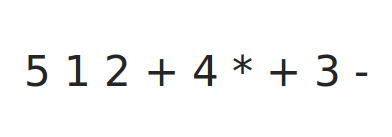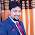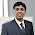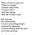# Postfix Evaluation using C++ StackAny equation in the form "5 + ((1 + 2) × 4) − 3" is called Infix expression. The postfix expression of this infix notation will be: "5 1 2 + 4 × + 3 −". It is also known as Reverse Polish notation.

#### Algorithm:

• Scan input expression from left to right
• If scanned input is an operand, push it into the stack
• If scanned input is an operator, pop out two values from stack. Then, perform operation between popped values and then push back the result into the stack.
• Repeat above two steps till all the characters are scanned.
• After all characters are scanned, there will be only one element in the stack, and this is the result of given expression.

#### C++ Implementation:

The following program evaluates a given postfix string. The numbers in inputs must be space separated:

```/*
* Postfix Evaluation
* Language: C++
*/

#include <stdio.h>
#include <iostream>
#include <stdlib.h>
#include <stack>
#include <string.h>

using namespace std;

bool isOperator(char ch)
{
if (ch=='+' || ch=='-' || ch=='*' || ch=='/')
return true;
else
return false;
}

int performOperation(int op1, int op2, char op)
{
int ans;
switch(op){
case '+':
ans = op2 + op1;
break;
case '-':
ans = op2 - op1;
break;
case '*':
ans = op2 * op1;
break;
case '/':
ans = op2 / op1;
break;
}
return ans;
}

int main()
{
char exp, buffer;
int i,op1, op2, len, j, x;
stack<int> s;
printf("Enter a Postfix Expression: ( e.g. 23 34 * )\n");
gets(exp);
len = strlen(exp);
j = 0;
for(i=0; i<len;i++){

if(exp[i]>='0' && exp[i]<='9'){
buffer[j++] = exp[i];
}
else if(exp[i]==' '){
if(j>0){
buffer[j] = '\0';
x = atoi(buffer);
s.push(x);
j = 0;
}
}

else if(isOperator(exp[i])){
op1 = s.top();
s.pop();
op2 = s.top();
s.pop();
s.push(performOperation(op1, op2, exp[i]));
}
}

printf("Answer is %d\n", s.top());

return 0;
}
```
Share:

#### 4 comments :

1.2.http://codingloverlavi.blogspot.in/2015/01/expression-evaluation-using-stacks.html
a more detailed solution using Stacks and LinkedList, works for a wide range of input

3.4.Why we use postfix evaluation in C++ ?? Give reason.

Spam comments will be deleted. :)